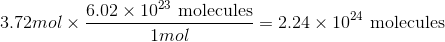# GED Science : Matter and Moles

## Example Questions

### Example Question #1 : Solids, Liquids, And Gases

Molecules are constantly moving. In which state of matter do molecules move the fastest?

Solids

Molecules move at the same rate in both gases and liquids

Liquids

Molecules move at the same rate in all three states of matter

Gases

Gases

Explanation:

The movement of particles is important in differentiating the states of matter. Solids have the lowest molecular energy; their molecules barely vibrate and are generally trapped in lattice structures. Molecules in liquids are able to move more freely, resulting in the fluidity of the liquid state. Gas molecules have even more freedom of movement, allowing the gas to expand to fill a space.

Consider that energy (such as heat) must be added to convert a solid to a liquid or a liquid to a gas. That energy goes to make the molecules move faster and increase the temperature of the compound. Gases require the most energy (consider boiling water), and will have the fastest moving molecules.

### Example Question #1 : Chemistry

Atomic mass values: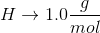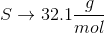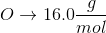Convert the following mass to moles: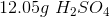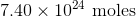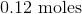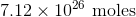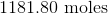Explanation:

To convert grams to moles, use the molecular weight of the molecule. This is found by adding the mass of each atom.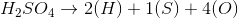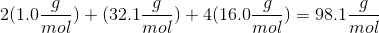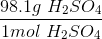Now we can use the molecular mass to convert the given mass in the question to moles. Make sure to align the values so that the units cancel out.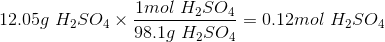### Example Question #2 : Chemistry

How many molecules are in 3.72mol of fluorine?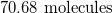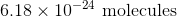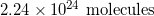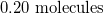Explanation:

Convert from moles to molecules using Avogadro's number: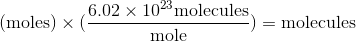Use the moles given in the question to solve.